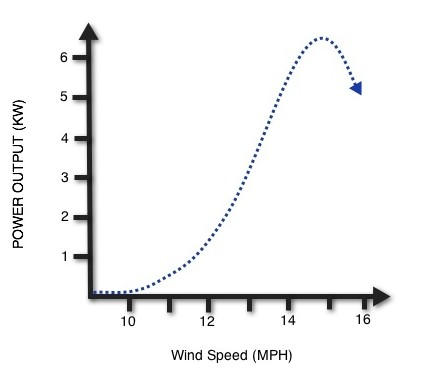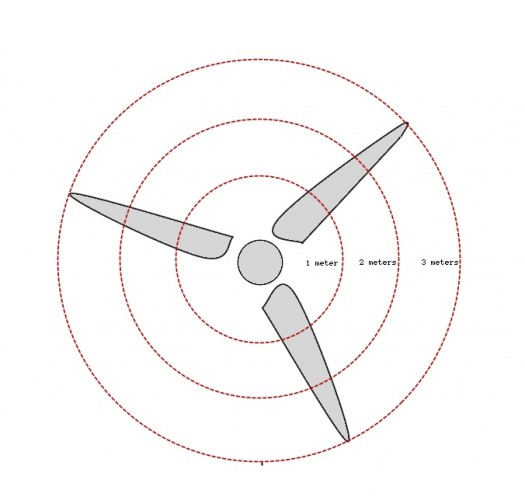# Wind Turbine Speed

An anemometer is a device used to measure wind speed.

Anemometers are often attached to wind turbines to control the start-up mechanism of wind turbines in low wind speeds, and also the shutting down of wind turbines in dangerously strong winds.

Wind speed is a contributing factor to the energy output potential of a wind turbine.

The greater the wind speed, the greater the energy output, assuming everything else is kept unchanged.

Wind speed has an approximately cubic relationship with energy output.

So, for example, if you were to double the wind speed, you would increase the power output by 8 times, it is easy to see this relationship in the graph provided below.Notice from the graph that at very low wind speeds the power output is near zero.  This is because all wind turbines have a distinct start-up speed and a cut-in speed.  The start-up speed is the minimum wind speed needed for the rotor and the blades to begin spinning, this low rotational speed will not provide any usable electric power.

The more important, cut-in speed, is the wind speed at which the turbine generator will begin to produce electricity.  This is a crucial piece of information to understand about wind turbine generators.  Just because the rotor and the blades are spinning, it does not mean that the generator is producing power.  At low wind and rotational speeds the turbine generator will produce no power until the wind speeds reach the required cut-in speed for that particular wind turbine.

The furling speed is the wind speed at which a turbine generator will shut off and stop generating power, usually to prevent damage to the turbine in cases of extraordinarily high wind speeds.

The graph above is a generic graph of no particular wind turbine generator, but still says a lot about the relationship between wind speed and power output.  In this particular graph the cut-in speed of the turbine is around 11 mph and the furling speed is when the output begins to decline around 15 mph.

Wind Turbine Tip Speed

The wind turbine tip speed is a measurement of how fast the end tip of a wind turbine blade is moving.

Every unique wind turbine has a different optimum blade speed that produce the highest amount of electrical power during operation.

There are two different speed measurements used for the speed of a wind turbine blades: linear speed, and angular speed.

Linear speed

Linear speed is the measurement of a length traveled during a unit of time.

For example riding a bike down the street at a speed of 15 miles/hour.

The linear speed of the wind turbine varies with the blade length, and also varies at different points on the same blade.

Here’s why:

Linear Speed of Blade = distance traveled / unit of time

Since the distance is measured as the circumference:

Linear Speed of Blade  = circumference / unit of time

= (2 x Pi x Radius) / unit of time

Since the radius is the longest at the tip of the turbine it is the point of the blade with the highest linear speed.  As the radius of the blade decreases at different points closer to the center rotor, the linear speed decreases.

Let’s take a look at the diagram below and find the linear speed for blades that make a complete rotation every second.  Each red circle represents a different point on the blades at: 1 meter from the center, 2 meters and 3 meters.  The Linear speed at each point on the blade is:

1 Meter = (2 x 3.14 x 1 meter) / (one second) = 6.28 meters / second

2 Meter = (2 x 3.14 x 2 meter) / (one second) = 12.6 meters / seocnd

3 Meter = (2 x 3.14 x 3 meter) / (one second) = 18.8 meters / second

Notice that the linear speeds increase as you mover further away from the center of the wind turbine, resulting in the wind turbine tip speed having the fastest linear speed than any other point on the blade.Angular speed is the measurement of degrees traveled per unit of time.  For example the minute hand on a clock rotates at 360 degrees / hour.  It can also be measured in radians / hour.  Every point on the wind turbine blade has the same angular speed because each point rotates 360 degrees in the same unit of time.

From the diagram above the angular speed is the same for each red circle.

Wind Turbine Tip Speed Ratio (TSR) is the speed of the tip divided by the speed of the wind.  For example if the tip speed is 120 miles/hour and the wind speed is 20 miles/hour than the TSR = 120 / 20 = 6.

The optimum tip TSR depends on how many blades are used to operate the wind turbine:

2 Blades : optimum ratio is around 6

3 Blades : optimum ratio is around 5

4 Blades : optimum ratio is around 3

The TSR is important because if the TSR is too low then the wind turbine will not be efficiently producing elecricity and may even rotate too slow to produce any energy at all, or too slow that it stalls.

If the TSR is too high then the wind turbine might reach it’s furling speed which would cause the wind turbine to begin to produce decreasing margins of electricity and may even shut down to avoid mechanical failure.